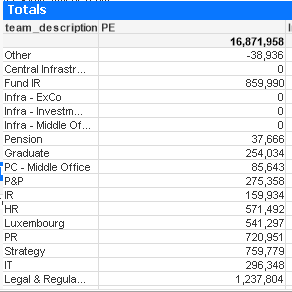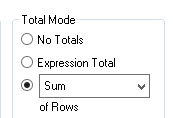# QlikView App Development

Discussion Board for collaboration related to QlikView App Development.

Highlighted
Contributor III

## Issues with 'sum of rows' / expression default summation

Hello

I have a table below, using sum of rowsWhen I change it to 'expression total' I get £16,732,021.

I actually just want one line in the table 'Total', so I remove the dimension 'team_description' and enter the calculated dimension showing 'Total'

and the number changes to below as though I am using 'expression default' as the summationHow can I replicate 'sum of rows' in my expression.  I think it has something to do with aggr and sum?  But it doesn't seem to work for me.  I attach my expression below  - but as you can see it is quite long!

Any help would be appreciated

Thanks

Paul

=If(
sum(aggr(((sum({\$<period = {">=\$(vStartPeriod_CY)<=\$(vEndPeriod_CY)"},
bud2 = {">0"},
team_description -= {'P&P','IT'},
Datasource = {'Actual'}, busarea = {PC}
>}
\$(vCurrency))

+

sum({\$<period = {">=\$(vStartPeriod_CY)<=\$(vEndPeriod_CY)"},
bud2 = {">0"},
team_description = {'P&P'},bud2 -= {200,260}, bud2_compen2 -={'Office Costs'},
Datasource = {'Actual'}, busarea = {PC}
>}
\$(vCurrency))

+

sum({\$<period = {">=\$(vStartPeriod_CY)<=\$(vEndPeriod_CY)"},
bud2 = {">0"},
team_description = {'IT'}, bud2_compen2 -={'Office Costs'},
Datasource = {'Actual'}, busarea = {PC}
>}
\$(vCurrency))
)
*
PE_Alloc%),team_description)) <> 0,

/////////////////////////////////////////////////////////////////////////////////

((
sum(aggr(((sum({\$<period = {">=\$(vStartPeriod_CY)<=\$(vEndPeriod_CY)"},
bud2 = {">0"},
team_description -= {'P&P','IT'},
Datasource = {'Actual'}, busarea = {PC}
>}
\$(vCurrency))

+

sum({\$<period = {">=\$(vStartPeriod_CY)<=\$(vEndPeriod_CY)"},
bud2 = {">0"},
team_description = {'P&P'},bud2 -= {200,260}, bud2_compen2 -={'Office Costs'},
Datasource = {'Actual'}, busarea = {PC}
>}
\$(vCurrency))

+

sum({\$<period = {">=\$(vStartPeriod_CY)<=\$(vEndPeriod_CY)"},
bud2 = {">0"},
team_description = {'IT'}, bud2_compen2 -={'Office Costs'},
Datasource = {'Actual'}, busarea = {PC}
>}
\$(vCurrency))
)
*
PE_Alloc%),team_description))
)
/
(
sum(aggr(((sum({\$<period = {">=\$(vStartPeriod_CY)<=\$(vEndPeriod_CY)"},
bud2 = {">0"},
team_description -= {'P&P','IT'},
Datasource = {'Actual'}, busarea = {PC}
>}
\$(vCurrency))

+

sum({\$<period = {">=\$(vStartPeriod_CY)<=\$(vEndPeriod_CY)"},
bud2 = {">0"},
team_description = {'P&P'},bud2 -= {200,260}, bud2_compen2 -={'Office Costs'},
Datasource = {'Actual'}, busarea = {PC}
>}
\$(vCurrency))

+

sum({\$<period = {">=\$(vStartPeriod_CY)<=\$(vEndPeriod_CY)"},
bud2 = {">0"},
team_description = {'IT'}, bud2_compen2 -={'Office Costs'},
Datasource = {'Actual'}, busarea = {PC}
>}
\$(vCurrency))
)
*
PE_Alloc%),team_description))

+

sum(aggr(((sum({\$<period = {">=\$(vStartPeriod_CY)<=\$(vEndPeriod_CY)"},
bud2 = {">0"},
team_description -= {'P&P','IT'},
Datasource = {'Actual'}, busarea = {PC}
>}
\$(vCurrency))

+

sum({\$<period = {">=\$(vStartPeriod_CY)<=\$(vEndPeriod_CY)"},
bud2 = {">0"},
team_description = {'P&P'},bud2 -= {200,260}, bud2_compen2 -={'Office Costs'},
Datasource = {'Actual'}, busarea = {PC}
>}
\$(vCurrency))

+

sum({\$<period = {">=\$(vStartPeriod_CY)<=\$(vEndPeriod_CY)"},
bud2 = {">0"},
team_description = {'IT'}, bud2_compen2 -={'Office Costs'},
Datasource = {'Actual'}, busarea = {PC}
>}
\$(vCurrency))
)
*
Infra_Alloc%),team_description))
)
)

*

sum(aggr(((sum({\$<period = {">=\$(vStartPeriod_CY)<=\$(vEndPeriod_CY)"},
bud2 = {">0"},
team_description -= {'P&P','IT'},
Datasource = {'Actual'}, busarea = {PC}
>}
\$(vCurrency))

+

sum({\$<period = {">=\$(vStartPeriod_CY)<=\$(vEndPeriod_CY)"},
bud2 = {">0"},
team_description = {'P&P'},bud2 -= {200,260}, bud2_compen2 -={'Office Costs'},
Datasource = {'Actual'}, busarea = {PC}
>}
\$(vCurrency))

+

sum({\$<period = {">=\$(vStartPeriod_CY)<=\$(vEndPeriod_CY)"},
bud2 = {">0"},
team_description = {'IT'}, bud2_compen2 -={'Office Costs'},
Datasource = {'Actual'}, busarea = {PC}
>}
\$(vCurrency))
)
*
PC_Alloc%),team_description))

,

////////////////////////////////////////

sum(aggr(((sum({\$<period = {">=\$(vStartPeriod_CY)<=\$(vEndPeriod_CY)"},
bud2 = {">0"},
team_description -= {'P&P','IT'},
Datasource = {'Actual'}, busarea = {PC}
>}
\$(vCurrency))

+

sum({\$<period = {">=\$(vStartPeriod_CY)<=\$(vEndPeriod_CY)"},
bud2 = {">0"},
team_description = {'P&P'},bud2 -= {200,260}, bud2_compen2 -={'Office Costs'},
Datasource = {'Actual'}, busarea = {PC}
>}
\$(vCurrency))

+

sum({\$<period = {">=\$(vStartPeriod_CY)<=\$(vEndPeriod_CY)"},
bud2 = {">0"},
team_description = {'IT'}, bud2_compen2 -={'Office Costs'},
Datasource = {'Actual'}, busarea = {PC}
>}
\$(vCurrency))
)
*
PC_Alloc%),team_description))*PE_PC_SPLIT

)
////////////////////////////////

+
sum(aggr(((sum({\$<period = {">=\$(vStartPeriod_CY)<=\$(vEndPeriod_CY)"},
bud2 = {">0"},
team_description -= {'P&P','IT'},
Datasource = {'Actual'}, busarea = {PC}
>}
\$(vCurrency))

+

sum({\$<period = {">=\$(vStartPeriod_CY)<=\$(vEndPeriod_CY)"},
bud2 = {">0"},
team_description = {'P&P'},bud2 -= {200,260}, bud2_compen2 -={'Office Costs'},
Datasource = {'Actual'}, busarea = {PC}
>}
\$(vCurrency))

+

sum({\$<period = {">=\$(vStartPeriod_CY)<=\$(vEndPeriod_CY)"},
bud2 = {">0"},
team_description = {'IT'}, bud2_compen2 -={'Office Costs'},
Datasource = {'Actual'}, busarea = {PC}
>}
\$(vCurrency))
)
*
PE_Alloc%),team_description))

Tags (2)
1 Solution

Accepted Solutions
New Contributor III

## Re: Issues with 'sum of rows' / expression default summation

A quick fix is probably to wrap your entire expression in a

SUM(

AGGR(

,team_description

)

)

When you do a SUM(AGGR(expression,team_description)), Qlik is creating a table behind the scenes with team_description as dimension and expression as the result, and then the outer SUM() will add them up according to your table dimensions. This is basically what you are doing by doing a sum of rows while having team_description as a dimension.

That said, your inner expression also has a lot of AGGRs on team_description as well, so it might be worth cleaning that up. You probably won't need to have nested AGGRs now that you know what it's doing behind the scenes, and without the nested AGGRs, you probably won't need to keep repeating the same 3 set analysis sums 7 times.

4 Replies
Valued Contributor

## Re: Issues with 'sum of rows' / expression default summation

Paul,

My immediate thought is that if your expression is that long then you probably need to do something in the script to tag up your data to make it simpler to define your sets/do your calculation. From that point possibly then easier to decode how to get the summation right, or it may drop away as an issue.

Is that an option?

Cheers,

Chris.

Valued Contributor

## Re: Issues with 'sum of rows' / expression default summation

Hi Paul.   Sum(Aggr(....), ListOfDimensions)   replicates the sum of rows but you have to aggregate the entire expression and not individual parts

New Contributor III

## Re: Issues with 'sum of rows' / expression default summation

A quick fix is probably to wrap your entire expression in a

SUM(

AGGR(

,team_description

)

)

When you do a SUM(AGGR(expression,team_description)), Qlik is creating a table behind the scenes with team_description as dimension and expression as the result, and then the outer SUM() will add them up according to your table dimensions. This is basically what you are doing by doing a sum of rows while having team_description as a dimension.

That said, your inner expression also has a lot of AGGRs on team_description as well, so it might be worth cleaning that up. You probably won't need to have nested AGGRs now that you know what it's doing behind the scenes, and without the nested AGGRs, you probably won't need to keep repeating the same 3 set analysis sums 7 times.

Contributor III

## Re: Issues with 'sum of rows' / expression default summation

Thanks all for your help.

The expression is really messy but when I used the format below as mentioned by Mike it worked.

Many thanks for all your help

SUM(

AGGR(